#### 期刊菜单

Research on WMR Control of Visual Servo with Quantitative Feedback
DOI: 10.12677/AIRR.2022.111002, PDF, HTML, XML, 下载: 71  浏览: 151

Abstract: A quantitative feedback control method based on super-twisting algorithm is proposed aiming at the trajectory tracking of image visual servo mobile robot with external disturbance and quantitative feedback. Firstly, based on super-twisting sliding mode control plan, the disturbance term is put into the high-order derivative of sliding mode to reduce the chattering in sliding mode control; Secondly, the limit of quantization errors are put into sliding mode switching function to suppress the influence of quantization on system stability; it is proved by Lyapunov function that the system can converge to a small region related to quantization parameters in finite time. Finally, by dynamically adjusting quantization parameters, the system can finally converge to zero. The effectiveness of the control plan is verified by simulation results.

1. 引言

2. 视觉伺服WMR系统

IBVS系统如图1所示，WMR在天花板具有固定摄像机的全局坐标系下，p为相机捕捉的特征点,将WMR前进速度v与转向角速度w看作控制输入， $\left(x\left(t\right),y\left(t\right)\right)$ 为特征点p全局坐标。

$\begin{array}{l}\stackrel{˙}{x}\left(t\right)=v\mathrm{cos}\theta \left(t\right)-wd\mathrm{sin}\theta \left(t\right)\\ \stackrel{˙}{y}\left(t\right)=v\mathrm{sin}\theta \left(t\right)+wd\mathrm{cos}\theta \left(t\right)\\ \stackrel{˙}{\theta }\left(t\right)=w\end{array}$ (1)

IBVS系统为：

$\stackrel{˙}{P}\left(t\right)=\frac{1}{z\left(t\right)}D\left(t\right)u+d\left(t\right)$ (2)

$D\left(t\right)=\left[\begin{array}{cc}{h}_{11}-{x}_{p}\left(t\right){h}_{31}& {h}_{12}-{x}_{p}\left(t\right){h}_{32}\\ {h}_{21}-{y}_{p}\left(t\right){h}_{31}& {h}_{22}-{y}_{p}\left(t\right){h}_{32}\end{array}\right]\left[\begin{array}{cc}\mathrm{cos}\theta \left(t\right)& -d\mathrm{sin}\theta \left(t\right)\\ \mathrm{sin}\theta \left(t\right)& d\mathrm{cos}\theta \left(t\right)\end{array}\right]$ (3)

$z\left(t\right)$ 为深度， $D\left(t\right)$ 是可逆的  $P\left(t\right)={\left({x}_{p}\left(t\right),{y}_{p}\left(t\right)\right)}^{\text{T}}$ 为图像系坐标， $d\left(t\right)={\left({d}_{x}\left(t\right),{d}_{y}\left(t\right)\right)}^{\text{T}}$ 为模型不确定、参数不确定和外界扰动的总和，WMR在图像系下的期望轨迹为 ${P}_{r}={\left({x}_{r},{y}_{r}\right)}^{\text{T}}$ 图像系下的误差定义为

$e\left(t\right)={P}_{r}-P\left(t\right)$ (4)

$s=e\left(t\right)+c{\int }_{0}^{t}e\left(t\right)\text{d}\tau$ (5)

$c>0$ 为待设定参数，对(5)求导可得

$\stackrel{˙}{s}=-\frac{1}{z\left(t\right)}D\left(t\right)u+ce\left(t\right)-d\left(t\right)$ (6)

$u=z\left(t\right)D{\left(t\right)}^{-1}\left\{ce\left(t\right)+{k}_{1}{|s|}^{\frac{1}{2}}sign\left(s\right)+{k}_{2}{\int }_{0}^{t}sign\left(s\right)\text{d}\tau \right\}$ (7)

(6)改写为

$\stackrel{˙}{s}=-{k}_{1}{|s|}^{\frac{1}{2}}sign\left(s\right)-{k}_{2}{\int }_{0}^{t}sign\left(s\right)\text{d}\tau +d\left(t\right)$ (8)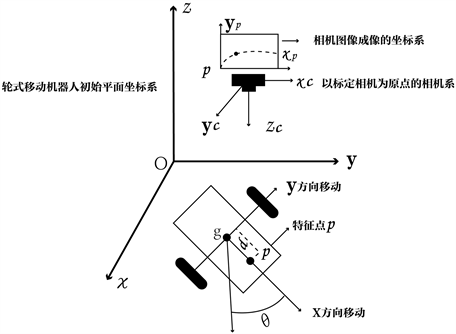Figure 1. IBVS system description

3. 量化反馈STSMC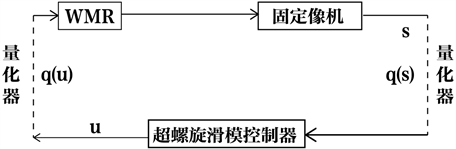Figure 2. Quantitative feedback visual servo closed-loop system

$q\left(s\right)=\mu ×round\left(\frac{s}{\mu }\right)$ (9)

$\mu >0$ 为量化参数， $round\left(\text{ }\right)$ 表示就近取整运算，量化误差为

$q\left(s\right)-s={e}_{qs}$ (10)

$|{e}_{qs}|=|q\left(s\right)-s|\le \frac{\mu }{2}$ (11)

$\stackrel{˙}{s}=-{k}_{1}{|q\left(s\right)|}^{\frac{1}{2}}sign\left(q\left(s\right)\right)-{k}_{2}{\int }_{0}^{t}sign\left(q\left(s\right)\right)\text{d}\tau +d\left(t\right)$ (12)

${|q\left(s\right)|}^{\frac{1}{2}}={|{e}_{qs}+s|}^{\frac{1}{2}}\le {|{e}_{qs}|}^{\frac{1}{2}}+{|s|}^{\frac{1}{2}}\le {|s|}^{\frac{1}{2}}+\sqrt{\frac{\mu }{2}}$ (13)

$|s|\ge \frac{\mu }{2}$$sign\left(q\left(s\right)\right)=sign\left(s\right)$，当 $|s|\le \frac{\mu }{2}$$q\left(s\right)=0$，将(12)改写为

$\left\{\begin{array}{l}\stackrel{˙}{s}=-{k}_{1}{|s|}^{\frac{1}{2}}sign\left(s\right)+f\left({e}_{qs}\right)+r\\ \stackrel{˙}{r}=-{k}_{2}sign\left(s\right)+\stackrel{˙}{d}\left(t\right)\end{array}$ (14)

$\left\{\begin{array}{l}{k}_{1}>2\\ {k}_{2}>\frac{{k}_{1}^{3}+{\eta }^{2}\left(4{k}_{1}-8\right)}{{k}_{1}\left(4{k}_{1}-8\right)}\end{array}$ (15)

$\xi =\left[\begin{array}{c}{|s|}^{\frac{1}{2}}sign\left(s\right)\\ {k}_{2}{\int }_{0}^{t}sign\left(s\right)\text{d}\tau +d\left(t\right)\end{array}\right]$ (16)

$\Omega \left(\mu \right)=\frac{\sqrt{{\lambda }_{\mathrm{max}}\left(P\right)}}{\sqrt{{\lambda }_{\mathrm{max}}\left(P\right)}}\frac{{k}_{1}\sqrt{\frac{\mu }{2}}\sqrt{{p}_{11}^{2}+{p}_{12}^{2}}}{\left(1-\omega \right){\lambda }_{\mathrm{min}}\left(Q\left(\eta \right)\right)}$ (17)

$\begin{array}{c}\stackrel{˙}{\xi }=\left[\begin{array}{c}\frac{1}{2|{\xi }_{1}|}\left(-{k}_{1}{|s|}^{\frac{1}{2}}sign\left(s\right)-{k}_{2}{\int }_{0}^{t}sign\left(s\right)\text{d}\tau +f\left({e}_{qs}\right)\right)\\ {k}_{2}sign\left(s\right)+\stackrel{˙}{d}\left(t\right)\end{array}\right]\\ =\frac{1}{|{\xi }_{1}|}\left[\begin{array}{cc}-\frac{{k}_{1}}{2}& \frac{1}{2}\\ -{k}_{2}+\delta \left(t\right)& 0\end{array}\right]\xi +\frac{1}{|{\xi }_{1}|}\left[\begin{array}{c}f\left({e}_{qs}\right)\\ 0\end{array}\right]\\ =\frac{1}{|{\xi }_{1}|}A\xi +\frac{1}{|{\xi }_{1}|}\left[\begin{array}{c}f\left({e}_{qs}\right)\\ 0\end{array}\right]\end{array}$ (18)

$V\left(\xi \right)={\xi }^{\text{T}}P\xi$ (19)

$P=\left[\begin{array}{cc}\frac{4{k}_{1}+{k}_{2}^{2}}{2}& \frac{-{k}_{2}}{2}\\ \frac{-{k}_{2}}{2}& 1\end{array}\right]$ (20)

$\begin{array}{c}\stackrel{˙}{V}\left(\xi \right)=\frac{1}{|{\xi }_{1}|}{\xi }^{\text{T}}\left({A}^{\text{T}}P+PA\right)\xi +\frac{1}{|{\xi }_{1}|}\left[f\left({e}_{qs}\right),0\right]P\xi \\ =-\frac{1}{|{\xi }_{1}|}{\xi }^{\text{T}}Q\left(\delta \left(t\right)\right)\xi +\frac{1}{|{\xi }_{1}|}f\left({e}_{qs}\right)\left[\begin{array}{cc}{p}_{11}& {p}_{12}\end{array}\right]\xi \end{array}$ (21)

$\stackrel{˙}{V}\left(\xi \right)\le -\frac{1}{|{\xi }_{1}|}{\xi }^{\text{T}}Q\left(\eta \right)\xi +\frac{1}{|{\xi }_{1}|}{k}_{1}\sqrt{\frac{\mu }{2}}\sqrt{{p}_{11}^{2}+{p}_{12}^{2}}‖\xi ‖$ (22)

$Q\left(\eta \right)=\left[\begin{array}{cc}{k}_{1}{k}_{2}+\frac{{k}_{2}^{3}}{2}-\frac{{k}_{2}^{2}}{4}-{\eta }^{2}& \frac{{k}_{2}}{2}-\frac{{k}_{2}^{2}}{2}\\ \frac{{k}_{2}}{2}-\frac{{k}_{2}^{2}}{2}& \frac{{k}_{2}}{2}-1\end{array}\right]$ (23)

${\lambda }_{\mathrm{min}}\left(Q\left(\eta \right)\right){‖\xi ‖}^{2}\le {\xi }^{\text{T}}Q\left(\eta \right)\xi \le {\lambda }_{\mathrm{max}}\left(Q\left(\eta \right)\right){‖\xi ‖}^{2}$ (24)

$|{\xi }_{1}|\le ‖\xi ‖$$‖\xi ‖\le \sqrt{\frac{V\left(\xi \right)}{{\lambda }_{\mathrm{min}}\left(P\right)}}$ 由此可得

$\begin{array}{c}\stackrel{˙}{V}\left(\xi \right)\le -\frac{1}{|{\xi }_{1}|}{\lambda }_{\mathrm{min}}\left(Q\left(\eta \right)\right){‖\xi ‖}^{2}+\frac{1}{|{\xi }_{1}|}{k}_{1}\sqrt{\frac{\mu }{2}}\sqrt{{p}_{11}^{2}+{p}_{12}^{2}}‖\xi ‖\\ \le -\frac{‖\xi ‖}{|{\xi }_{1}|}\left\{{\lambda }_{\mathrm{min}}\left(Q\left(\eta \right)\right)‖\xi ‖-{k}_{1}\sqrt{\frac{\mu }{2}}\sqrt{{p}_{11}^{2}+{p}_{12}^{2}}\right\}\\ \le -{\lambda }_{\mathrm{min}}\left(Q\left(\eta \right)\right)‖\xi ‖+{k}_{1}\sqrt{\frac{\mu }{2}}\sqrt{{p}_{11}^{2}+{p}_{12}^{2}}\\ \le -{\lambda }_{\mathrm{min}}\left(Q\left(\eta \right)\right)\left[\omega ‖\xi ‖-\left(1-\omega \right)‖\xi ‖\right]+{k}_{1}\sqrt{\frac{\mu }{2}}\sqrt{{p}_{11}^{2}+{p}_{12}^{2}}\end{array}$ (25)

$\forall ‖\xi ‖\ge {k}_{1}\sqrt{\frac{\mu }{2}}\frac{\sqrt{{p}_{11}^{2}+{p}_{12}^{2}}}{\left(1-\omega \right){\lambda }_{\mathrm{min}}\left(Q\left(\eta \right)\right)}$ 时有

$\stackrel{˙}{V}\left(\xi \right)\le \frac{-\omega {\lambda }_{\mathrm{min}}\left(Q\left(\eta \right)\right)}{{\lambda }_{\mathrm{max}}^{\frac{1}{2}}\left(P\right)}{V}^{\frac{1}{2}}\left(\xi \right)$ (26)

${t}_{f}=\frac{2\sqrt{{\lambda }_{\mathrm{min}}\left(P\right)}\left\{{V}^{\frac{1}{2}}\left(0\right)-{k}_{1}\sqrt{\frac{\mu }{2}}\frac{\sqrt{{\lambda }_{\mathrm{max}}\left(P\right)}\sqrt{{p}_{11}^{2}+{p}_{12}^{2}}}{\left(1-\omega \right){\lambda }_{\mathrm{min}}\left(Q\left(\eta \right)\right)}\right\}}{\omega {\lambda }_{\mathrm{min}}\left(Q\left(\eta \right)\right)}$ (27)

$\stackrel{˙}{s}=-\frac{1}{z\left(t\right)}D\left(t\right)q\left(u\right)+ce\left(t\right)-d\left(t\right)$ (28)

$\left\{\begin{array}{l}\stackrel{˙}{s}=-{k}_{1}{|s|}^{\frac{1}{2}}sign\left(s\right)+f\left({e}_{qs}\right)+{e}_{qu}+r\\ \stackrel{˙}{r}=-{k}_{2}sign\left(s\right)+\stackrel{˙}{d}\left(t\right)\end{array}$ (29)

$‖\xi ‖\le {\Omega }^{\ast }\left(\mu ,{\mu }^{\ast }\right)=\frac{\sqrt{{\lambda }_{\mathrm{max}}\left(P\right)}}{\sqrt{{\lambda }_{\mathrm{max}}\left(P\right)}}\frac{\left({k}_{1}\sqrt{\frac{\mu }{2}+}\frac{{\mu }^{*}}{2}\right)\sqrt{{p}_{11}^{2}+{p}_{12}}}{\left(1-\omega \right){\lambda }_{\mathrm{min}}\left(Q\left(\eta \right)\right)}$ (30)

4. 数值与仿真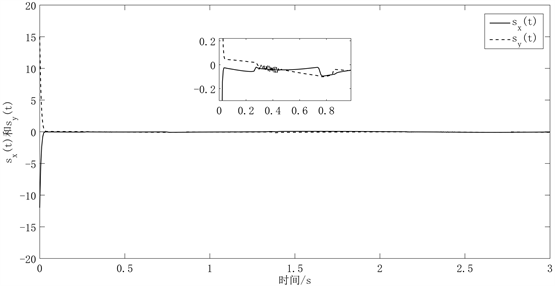Figure 3. Dynamic uniform quantization of the system state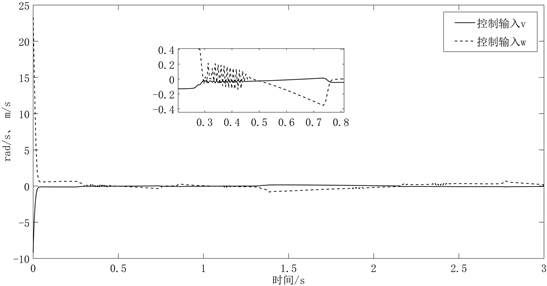Figure 4. Dynamic uniform quantization of control inputs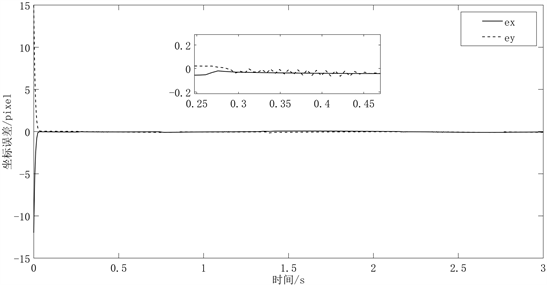Figure 5. Trajectory tracking errorFigure 6. Real trajectories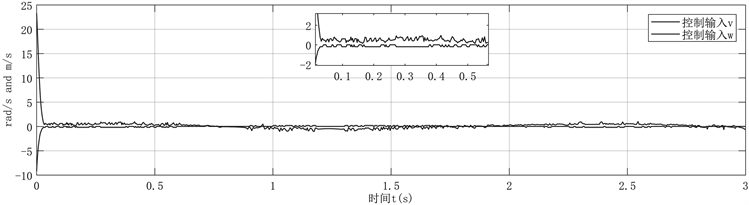Figure 7. The state of the system under static quantization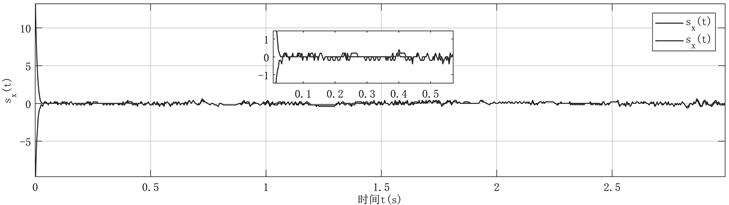Figure 8. Control input under static quantization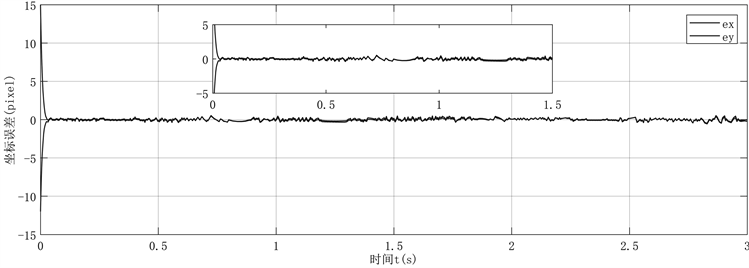Figure 9. Systematic error under static quantization

5. 总结

  李宝全, 徐壮, 冀东. 基于单应矩阵分解的轮式移动机器人视觉伺服轨迹跟踪[J]. 天津工业大学学报, 2020, 39(3): 61-68.  Cheah, C.C., Hou, S.P., Yu, Z., et al. (2010) Adaptive Vision and Force Tracking Control for Robots with Constraint Uncertainty. IEEE/ASME Transactions on Mechatronics, 15, 389-399. https://doi.org/10.1109/TMECH.2009.2027115  Liu, Y.H., Wang, H., Wang, C., et al. (2006) Uncalibrated Visual Servoing of Robots Using a Depth-Independent Interaction Matrix. IEEE Transactions on Robotics, 22, 804-817. https://doi.org/10.1109/TRO.2006.878788  Li, S., Ghasemi, A., Xie, W., et al. (2018) An Enhanced IBVS Controller of a 6DOF Manipulator Using Hybrid PD-SMC Method. International Journal of Control Automation and Systems, 16, 844-855. https://doi.org/10.1007/s12555-016-0720-4  张德伟, 刘海涛, 王仙业, 肖聚亮. 一种足式移动机器人的混合视觉伺服控制方法[J/OL]. 机械科学与技术: 1-10. https://doi.org/10.13433/j.cnki.1003-8728.20200527  陈勇, 刘哲, 乔健, 卢清华, 谢永芳. 轮式机器人移动过程中滑模控制策略的研究[J]. 控制工程, 2021, 28(5): 963-970.  方文华. 采用改进粒子群算法优化的两关节移动机器人滑模控制研究[J]. 中国工程机械学报, 2019, 17(6): 506-509+514.  陈杰, 刘宜成, 王宏, 涂海燕. 图像视觉伺服的无人机固定时间滑模控制[J]. 电光与控制, 2021, 28(3): 24-30.  李军, 廖宇新, 李珺. 三维自适应有限时间超螺旋滑模制导律[J]. 系统工程与电子技术, 2021, 43(3): 779-788.  薛艳梅, 郝立颖. 量化参数不匹配的线性系统监督滑模控制设计[J]. 计算机工程与应用, 2015, 51(15): 22-27.  张乐乐, 苏前敏. 具有不匹配量化的非线性系统自适应滑模控制[J]. 电子科技, 2020, 33(12): 38-43.  Sun, H., Hou, L., Zong, G., et al. (2018) Fixed-Time Attitude Tracking Control for Spacecraft with Input Quantization. IEEE Transactions on Aerospace and Electronic Systems, 55, 124-134. https://doi.org/10.1109/TAES.2018.2849158  Crochiere, R.E.A (2014) Mid-Rise/Mid-Tread Quantizer Switch for Improved Idle-Channel Performance in Adaptive Coders. Bell System Technical Journal, 57, 2953-2955. https://doi.org/10.1002/j.1538-7305.1978.tb02185.x  冯昱澍, 刘昆, 冯健. 航天器姿态跟踪有限时间自适应积分滑模控制[J]. 电子科技大学学报, 2021, 50(4): 527-534.